# News

## MS Math April 25-29, 20226th Grade Math – Mrs. Evans

This week we will finish Chapter 10.   We will be able to calculate the area and perimeter of most simple forms – squares, rectangles, parallelograms, triangles, trapezoids and regular polygons.  We can use these simple shapes to model composite shapes in order to find the area of these figures.  By knowing how to find the area of simple shapes we can use our critical thinking to apply it to many shapes we see.  Here is a video showing how to apply simple shape formula to find the area of composite shapes to find the area and the perimeter:

Math Grade Math – Mrs. Evans and Mrs. VonFeldt

This week, the 7th graders will be finishing Module 10. In Module 10, we have understood that when we want to study a population of something, we can study a random sample of that population, evaluate the data, and make inferences (predictions or educated guesses).  This is very useful in the real world.  This is an overall picture of how the process works: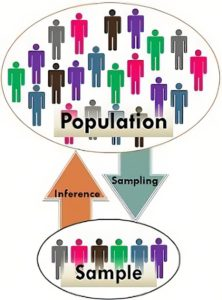Algebra – Mrs. VonFeldt

This week we will learn the last two strategies for solving quadratic equations: Completing the Square and the Quadratic Formula. The Quadratic Formula can be used for any quadratic equation, however, it is particularly useful when the “a” “b” and “c” terms are not integers. It is so useful in fact, that it is worth spending the time to memorize it. (see the song below). We will spend time this week reviewing all the strategies we have learned and determining which one is the best for a particular equation. There will be a test on Chapter 9 next week.

QUADRATIC EQUATION: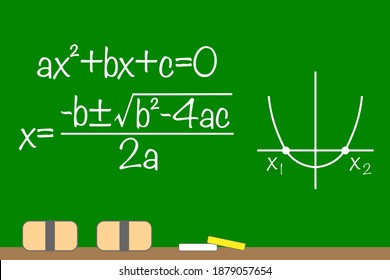## MS Math April 18-22, 20226th Grade Math – Mrs. Evans

This week we will continue studying geometry in Chapter 10.  We have been investigating shape areas and creating formulas that we can use to calculate area.  We have already found the formula for squares, rectangles, parallelograms and triangles.  This week we will find formulas for trapezoids and regular polygons.  We will use these formulas to find the area of composite figures, which are figures that are made up of more than one simpler shape.

Math Grade Math – Mrs. Evans and Mrs. VonFeldt

The 7th graders are studying statistics, working lessons in Module 10.  We are looking at populations of things we are interested in studying by sampling them.  We want to have random, unbiased samples surveyed.  If we have this, we can assume that we can make reasonable inferences (predictions) about the population.  We are learning to plot data so that we can make predictions.  We are using dot plots and box plots.  Dot plots are easy to create while box plots are a little more complicated.  Here is a video showing how to create these.

8th Grade Math – Mrs. Ernest

The 8th graders will be practicing solving multistep equations. To work through these problems, students will first need to copy down the problem, then circle and box different terms.Algebra – Mrs. VonFeldt

Students are graphing parabolas and solving quadratic functions. We will begin to learn multiple strategies to solve equations. We will use the vertex, axis of symmetry, and y-intercept to help them quickly graph a function. All of these skills are particularly helpful in analyzing real-world situations relating to sports, physics, architecture, nature, and more!## MS Math Weeks of March 21-25 & March 28-April 1Karen Evans Photography

Happy Spring!

This post covers the next two weeks until spring break.  Have a great spring break!

6th Grade Math – Mrs. Evans

This week we will finish Chapter 8 with a review and test, however we will continue our study of algebra.  In Chapter 9 we will see how two variables relate to each other in a proportional equation.  One variable is dependent on the other.  We can set up equations, tables and graphs to show proportional (or direct) relationship between two variables.  We will be investigating these skills, finishing up before spring break next week.

We can understand independent and dependent variables by viewing this spring-like picture below.  We can set up a relationship between the amount of water we give our plants and the status of the leaves: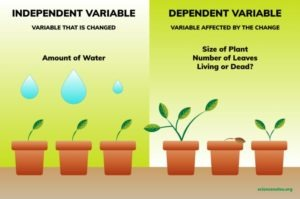Math Grade Math – Mrs. Evans and Mrs. VonFeldt

The 7th graders will continue their study of geometry, practicing finding the surface area and volume of prisms and compound solids.  One very important skill we have been practicing in this module is using formulae.  We will finish Module 9 this week, do a review and test early next week.  We will finish up before spring break with a geometry project.  Here is a video to help with finding the volume of prisms:

8th Grade Math – Mrs. Ernest

The 8th graders will be practicing their understanding of volume and area of complex figures. Students will be building paper villages and calculating the surface area and volume of their entire village!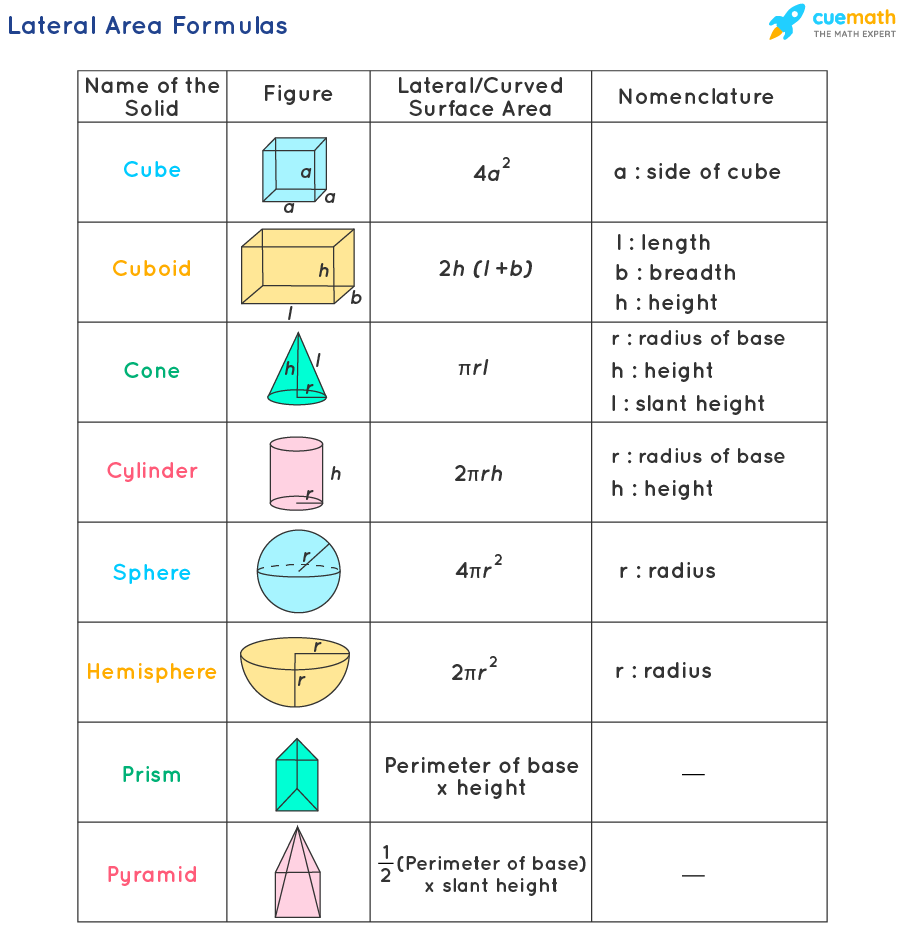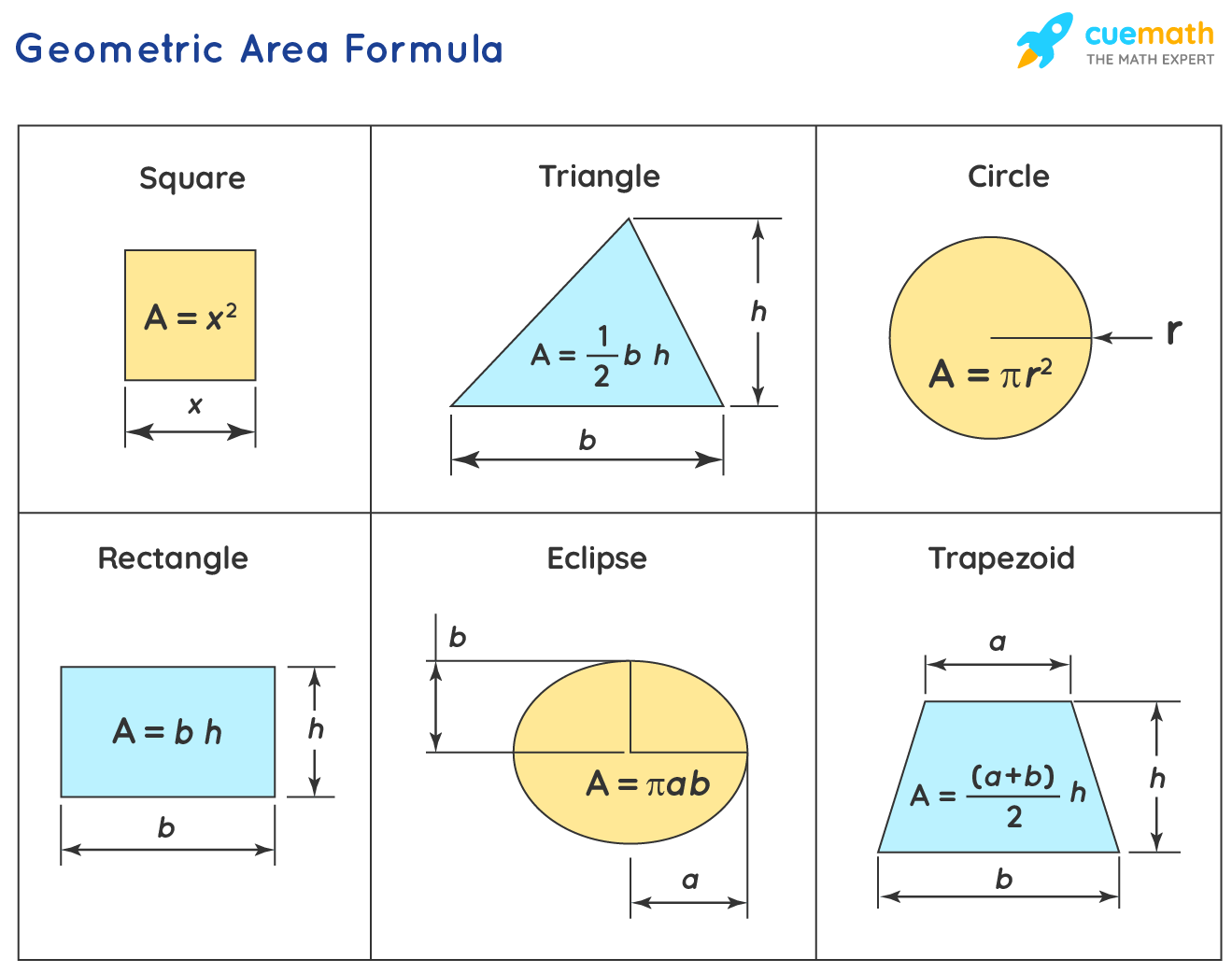Algebra – Mrs. VonFeldt

This week students are learning to factor trinomials (quadratic expressions). Quadratic equations are very useful in real life. Some examples include: area calculations for plots of land, calculating speed, height, or distance involving projectile motion, and genetics. We will model factoring with tiles and then look for patterns to help us develop an algorithm to factor many kinds of quadratics. The following Khan Academy video is a helpful introduction to this concept:

## MS Math Feb 28-March 4, 20226th Grade Math – Mrs. Evans

This week we will finish Chapter 7 with a test on Tuesday or Wednesday.  We now have an excellent foundation to do some basic algebra.  Here is a video discussing many of the basic topics we have covered.

After we finish the test, we will extend our algebra skills learning about equations and how to solve them.

Math Grade Math – Mrs. Evans and Mrs. VonFeldt

The 7th grade has completed Module 8 and will test on the topics mid-week.  In this module we have learned about triangles, scale drawings and angle relationships.  We will move into Module 9, in which we will investigate circles.  For those wanting a little more help with the module 8 skills, please visit this area in KHAN ACADEMY:

8th Grade Math – Mrs. Ernest

The 8th graders will be revisiting graphing skills and working on Geometry concepts like Translating, Rotating, and Reflecting shapes mathematically! Students will review similar shapes as they work to graph and shift different polygons. As we move forward this week, students will be able to both describe the side lengths, angles, and types of transformations different shapes have gone through. On Friday, we will work on a tessellations project, inspired by the mathematical artwork of M.C. Escher!Algebra – Mrs. VonFeldt

Students continue to practice simplifying exponential expressions using all the rules of exponents. This week we will explore exponential growth and exponential decay functions. Some very important real world problems can be studied or explained using exponential models. Examples include compounded interest, population growth or decline, radioactive decay, and depreciation. We will use the exponential growth model (shown below) to compare the difference between compounding interest monthly versus yearly. The Chapter 7 Test will be early next week.The following video is a good summary of how to use exponential functions:

## MS Math – Feb 7-18, 20226th Grade Math – Mrs. Evans

This week we are continue working in Chapter 7.  Last week we learned about exponents and how to write and evaluate numeric expressions using the proper order of operations.   This order can be summed up with the numonic:  PEMDAS, which is shown below: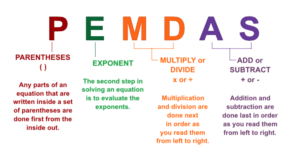This week we will begin writing algebraic expression and learning how to evaluate those expressions for given values of the variables.  Then we will be learning to simplify expressions by combining like terms.  These skills will be the basis for algebraic studies.

Math Grade Math – Mrs. Evans and Mrs. VonFeldt

Last week we began our study of geometry in Module 8.  We learned about scale drawings and will use proportions to solve for dimensions.  Proportions are useful in many areas of math.  We last encountered them when we were evaluating rates.  Proportions can in a number of ways, the most direct being CROSS MULTIPLICATION.  Here is a video that discusses solving proportions:

This week we will be making observations about triangles.  We will learn about the Triangle Inequality Theorem which summarizes how we can tell if 3 lines will create a triangle.  We will also learn about the angles of a triangle.  We will be using a great program called GEOGEBRA, which is a great place for investigation.  Here is a link to the program:

https://www.geogebra.org/

8th Grade Math – Mrs. Ernest

The 8th graders will be practicing their algebra skills as we solve for missing angles in triangles, complimentary and supplementary angle problems. Students will be able to identify like terms (terms with X or without X) and move numbers from one side of an equation to the other. We will have a quiz on these skills later in the week.

Algebra – Mrs. VonFeldt

We have finished our study of systems of equations and systems of inequalities. Students should be able to evaluate a system and determine the most effective method to solve. There will be a test on Chapter 6 on Wednesday.## MS Math Jan 31 – Feb 4, 2022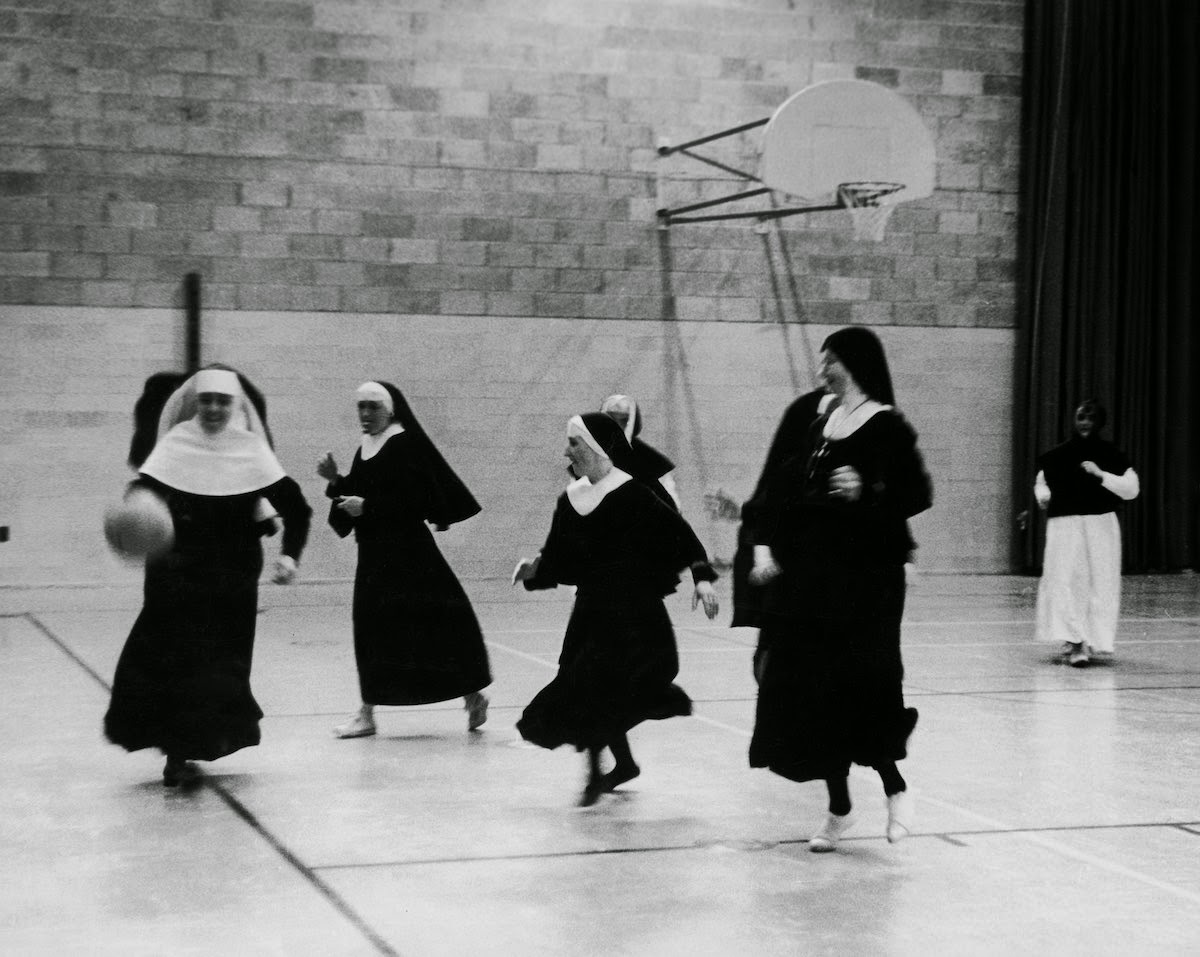Happy Catholic Schools Week!

We are grateful to be part of a long history of Catholic education!

6th Grade Math – Mrs. Evans

This week we will finish our study of unit conversions with a Chapter 6 test on Thursday.  In this chapter we have learned that units can help us to set up and solve problems.  Just like numbers, units can “cancel out” leaving the correct units if we set up our problem correctly.  Our test will also cover using rate, distance and time formulas.  While rate, distance and time are related in one way, we can rearrange the formula to solve for whatever of the three we are needing.  Here are the formulas in different forms:Math Grade Math – Mrs. Evans and Mrs. VonFeldt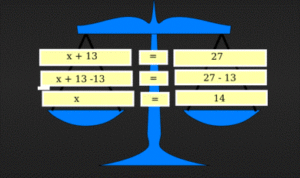This week we will conclude our algebra studies with a review on Monday and a test on Wednesday.  This topic is cumulative as each skill builds the foundation for the next.  It will cover expressions, equations and inequalities.  For students wanting to practice this week, IXL.COM (sections R, T and U) would be an excellent place to do so.  It provides immediate feedback and, when you scroll to the bottom of the page, explanation and examples of problems done correctly.  If you want more help, please reach out to Mrs. Evans or Mrs. VonFeldt.   Later in the week, we will begin our study of geometry with scale drawings.  Here is a link to IXL.         IXL | Learn 7th grade math

8th Grade Math – Mrs. Ernest

The 8th graders will be jumping into Chapter 5, which explores angles, triangles, and similarity! Students will learn how to use a protractor and learn the following terms:Algebra – Mrs. VonFeldt

This week, we are exploring what happens when you have a set of two equations graphed on the same axes. These are called systems of linear equations and the solution is the ordered pair where the two lines intersect, or a point that is on both lines. We will be learning three methods for solving systems of equations: Graphing, Substitution, and Elimination. Each method can be useful depending on how the equations are written. See the image below for a brief explanation of each strategy.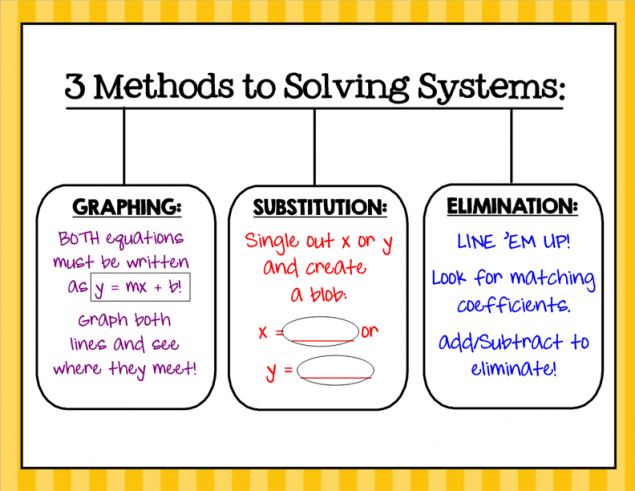## MS Math – Jan 24-286th Grade Math – Mrs. Evans

This week we will continue to learn about converting between units of measurement.  We will be working with both customary units and metric units.  By using conversion factors, we can convert between any two units regardless of the system.  Here is how we can use a conversion factor:The metric system is a base ten system so you can also make conversions with this method:Math Grade Math – Mrs. Evans and Mrs. VonFeldt

This week we will continue our study of algebra as we write, model and solve one and two step inequalities.  While the solution of an equation is a single value, the solution of an inequality is a range of numbers.  With inequalities, we can use the same logic as solving equations.  The DIFFERENCE between solving equations and inequalities, is that FOR INEQUALITIES, if you multiply or divide both sides by a negative number, you must reverse the inequality symbol for it to remain true.  Here are summaries on how to solve equations and inequalities:8th Grade Math – Mrs. Ernest

Last week, 8th graders perfected their ability to find whether or not fractions were proportional to one another using cross multiplication. This week, we will extend that learning by finding similar figures, solving for missing side lengths, and building our confidence with geometric word problems and graphing.

IXL review:
Level 1:
Level 2:
Level 3:
Unit Rates Bonus Practice:

Algebra – Mrs. VonFeldt

This week, we finished Chapter 5 and our study of inequalities. Students are now familiar with graphing inequalities in one or two variables. We will continue to practice solving and graphing equations as we begin to look at different ways to solve systems of equations.

## MS Math – Nov 29-Dec 3We hope you had a wonderful Thanksgiving!

6th Grade Math – Mrs. Evans

This week we will continue the study of ratios, rates and unit rates in Chapter 4. Rates and unit rates are very useful mathematical concepts that we use in day to day life, such as when we buy things (\$/packages) or maintain a safe speed in the car (miles/hour).  Take the opportunity to discuss as many of these situations that your family encounters this week!  Here is a video to help your student understand Ratios, Rates and Unit Rates:

Math Grade Math – Mrs. Evans and Mrs. VonFeldt

This week, we will do what we had planned to do last week – start Module 5!    We will learn about finding the percent change in different amounts.  I include this from last week because it did not get covered last week.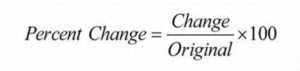We will be determining the markup and markdown on quantities using percentages.  Finding markup and markdown requires thinking logically.  Here is a video about percent change to introduce you to this useful concept.

We will use this concept in solving real world problems.  As a family you can call attention to all the places that we use percentages in day to day life; when we shop, eat out, leave a tip, pay taxes, make interest on our savings or pay interest on loans.  It is a great time to talk about these situations with your student!

8th Grade Math – Mrs. Ernest

The students are learning how to apply exponent rules to Scientific Notation this week! We will begin by learning the Scientific Notation format, then we will practice converting large and small numbers into Scientific Notation. Next, we will work backwards- turning a number written in the notation back to a large or small number. Students will learn how to multiply and divide Sci Notation numbers, and if we have time, we will begin to apply all of our learning about exponents to a new concept: Square Roots!

Algebra – Mrs. VonFeldt

This week, we will continue to practice writing and graphing linear equations. Students will learn how to express a line in point-slope form. This form is particularly helpful when you are given a slope and a random point on the line. Then, they will learn how to rewrite that equation into slope-intercept form or standard form. Students should be able to go back and forth between these three forms by rewriting the equation.

## MS Math – Nov 15-19, 20216th Grade Math – Mrs. Evans

This week we will finish with graphing rational numbers in Chapter 3 with a test on Wednesday.  We will begin to investigate ratios, rates and unit rates as we work into Chapter 4.  Here is a video about ratios and rates:

Math Grade Math – Mrs. Evans and Mrs. VonFeldt

This week, we will finish our study of proportional relationships with a heavy quiz on  Thursday.  We have learned a lot in this module and will be able to apply it throughout the rest of the year.  In Module 5 we will plunge into studying about percents and how to determine a percent change.  The percent change is defined as follows:We will be determining the markup and markdown on quantities using percents.  Here is a video about percent change to introduce you to this useful concept.

8th Grade Math – Mrs. Ernest

Algebra – Mrs. VonFeldt

There will be a Chapter 3 test on Thursday, November 18. It will cover graphing linear equations. In Chapter 4 we will look at ways to find the equation of a line when you have different pieces of information. How can you find an equation when you have two points? A point and a slope? A y-intercept and a point? Students are getting very familiar with the different representations of linear situations. They can now graph directly from an equation, create a table from an equation or graph, and find an equation directly from a graph.

## MS Math – Nov 8-12, 20216th Grade Math – Mrs. Evans

We will only have 3 days of math this week as Thursday is Veterans day and Friday the 6th graders will be doing a service project.

This week will explore graphing on the coordinate system.  We will graph ordered pairs of rational numbers and analyze some interesting things about them, like quadrants, line symmetry and distance between points.  Here is a picture of the coordinate plane:Math Grade Math – Mrs. Evans and Mrs. VonFeldt

This week, as we work in Module 4, we will continue to learn about how to write proportional equations, how to graph them, what they look like when on a graph and how to analyze a graph for information about the proportional relationship.  Here is a video from Khan Academy about writing proportional equations:

And here is another video from Khan Academy about graphing proportional equations:

8th Grade Math – Mrs. Ernest

Students have been learning about linear equations (y=mx+b). This unit we will transition to thinking about more complex equations, like quadratics! Students will be introduced to the exponent rules and learn more about algebra with exponents as we move forward.

Algebra – Mrs. VonFeldt

We are continuing to explore how linear functions can be written and graphed. This week we will look at proportional relationships. Proportional relationships are just linear functions that have a common ratio called the constant of proportionality, k. The graphs go through the origin. See the Khan Academy videos above in 7th grade for review.

This week we will also learn function notation. Function notation is a fancy algebraic way of writing “y”.The following Math Antics video is an excellent summary of what we have learned so far about linear functions:

© 2022 Assumption Catholic School | 2116 Cornwall Ave, Bellingham, WA 98225
Phone: 360.733.6133 | Fax: 360.647.4372
Email: theoffice@school.assumption.org
Dashboard | Web design and development by Olywebdev.com